StatLect

# Beta function

The Beta function is a function of two variables that is often found in probability theory and mathematical statistics (for example, as a normalizing constant in the probability density functions of the F distribution and of the Student's t distribution). We report here some basic facts about the Beta function.## Definition

The following is a possible definition of the Beta function:

Definition The Beta function is a functiondefined as follows:whereis the Gamma function.

While the domain of definition of the Beta function can be extended beyond the setof couples of strictly positive real numbers (for example to couples of complex numbers), the somewhat restrictive definition given above is more than sufficient to address all the problems involving the Beta function that are found in these lectures.

## Integral representations

The Beta function has several integral representations, which are sometimes also used as a definition of the Beta function, in place of the definition we have given above. We report here two often used representations.

### Integral between zero and infinity

The first representation involves an integral from zero to infinity:Proof

Given the definition of the Beta function as a ratio of Gamma functions (see above), the equality holds if and only if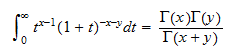or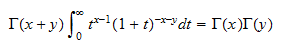That the latter equality indeed holds is proved as follows: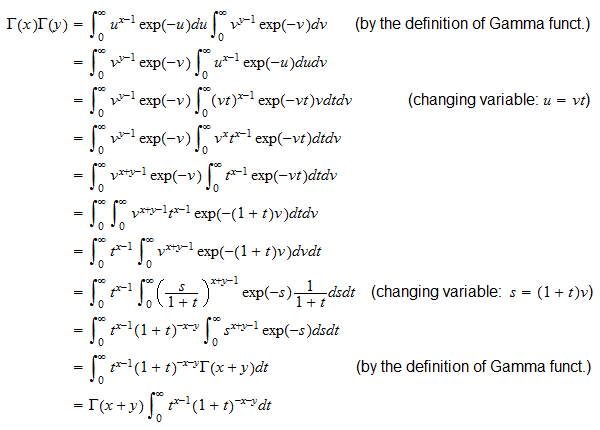### Integral between zero and one

Another representation involves an integral from zero to one:Proof

This can be obtained from the previous integral representation:by performing a change of variable. The change of variable isBefore performing it, note thatand thatFurthermore, differentiating the previous expression we obtainWe are now ready to perform the change of variable: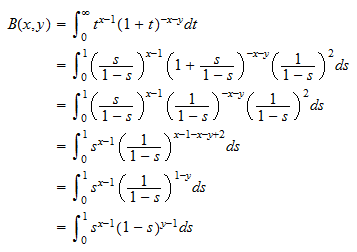Note that the two representations above involve improper integrals that converge ifand: this might help you to see why the arguments of the Beta function are required to be strictly positive.

## More details

The following sections contain more details about the Beta function.

### Incomplete Beta function

The integral representation of the Beta functioncan be generalized by substituting the upper bound of integration () with a variable ():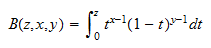The functionthus obtained is called incomplete Beta function.

## Solved exercises

Below you can find some exercises with explained solutions.

### Exercise 1

Compute the following product:whereis the Gamma function andis the Beta function.

Solution

We need to write the Beta function in terms of Gamma functions: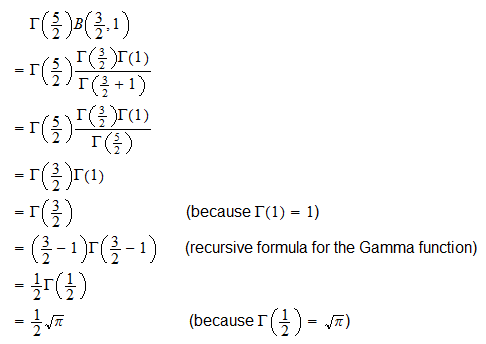where we have used several elementary facts about the Gamma function, that are explained in the lecture entitled Gamma function.

### Exercise 2

Compute the following ratiowhereis the Beta function.

Solution

This is achieved by rewriting the numerator of the ratio in terms of Gamma functions and using the recursive formula for the Gamma function: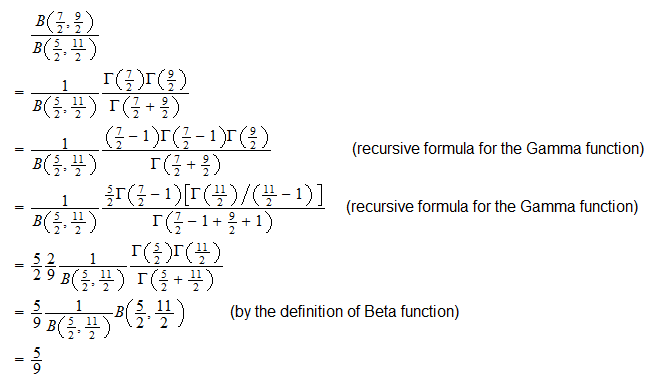### Exercise 3

Compute the following integral:Solution

We need to use the integral representation of the Beta function: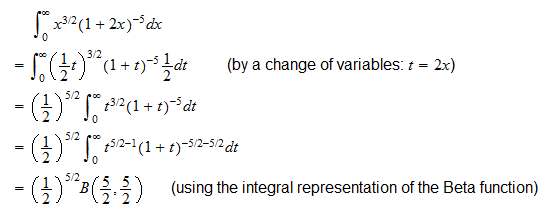Now, write the Beta function in terms of Gamma functions: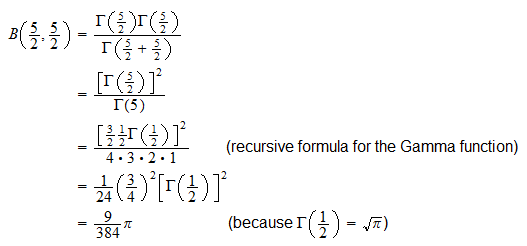Substituting this number into the previous expression for the integral, we obtainIf you wish, you can check the above result by using the following MATLAB commands:

`syms x`

`f=(x^(3/2))*((1+2*x)^-5)`

`int(f,0,Inf)`

The book

Most of the learning materials found on this website are now available in a traditional textbook format.

Glossary entries
Share